## Square

Positive charge Q is located in all vertices of a square with side length of a.

a) Determine the electric field intensity in the centre of the square.

b) What charge q do we have to place at the centre of the square so that the overall forces acting on every charge were zero?

• #### a) Hint: Intensity And Potential Of Electric Field

All charges have the same value and distance from the centre. What will thus apply for electric field intensity vectors’ size?

The potential in the centre of the square can be obtained by adding up the potentials of all electric fields what the point charges create.

• #### a) Solution: Electric Field Intensity

The size of electric intensity is directly proportional to the size of the charge creating the electric field and indirectly proportional to the square of the distance between the charge and the point where we determine the intensity.

All charges are of the same size and have the same distance between them and the centre of the square. The size of electric intensity of all fields is thus the same.

The direction of the vector of the electric intensity is the same as the direction of the electric force that would act on a positive charge in the given point. Since the charges in the vertices are positive, the electric intensity will point away from them. If we draw a picture, it is obvious that vectors of electric intensity of fields of opposite charges have opposite directions. Since they also have the same size, they will cancel out.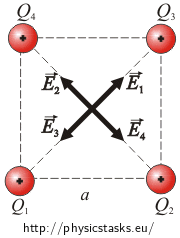The total electric field intensity will thus be zero.

• #### a) Analysis: Potential In the Square Centre

The total electric field potential in the centre of the square can be obtained by adding up the potential of all electric fields caused by all the charges. The electric field potential of a point charge is directly proportional to the size of the charge and indirectly proportional to the distance between the charge and the point where we determine the potential. Since all charges are of the same size and have the same distance from the centre, the potential caused by each of them at the centre of the square is the same.

• #### a) Solution: Potential In the Square Centre

The total potential in the centre of the square is equal to the sum of potentials of every charge.

The following relation applies for electric potential at distance r caused by charge Q:

$\varphi\,=\, \frac{1}{4 \pi \varepsilon_0} \frac{Q}{r}\,.$

Since all charges have the same value and are the same distance from the centre of the square, the potential is always the same. Charges are half the diagonal distant from the centre of the square. That distance is $$\frac{a \sqrt{2}}{2}$$. The potential of every field is equal to:

$\varphi\,=\, \frac{1}{4 \pi \varepsilon_0}\, \frac{Q}{\frac{a \sqrt{2}}{2}}\,=\,\frac{1}{4 \pi \varepsilon_0}\, \frac{2Q }{a\sqrt{2} }\,.$

We will multiply the denominator and the numerator by the square root of 2 to remove the root from the denominator.

$\varphi\,=\,\frac{1}{4 \pi \varepsilon_0}\, \frac{2\sqrt{2}Q }{2a }\,=\,\frac{1}{4 \pi \varepsilon_0}\, \frac{\sqrt{2}Q }{a }$

We will obtain the total potential by adding up all potentials of every field, i.e. four times the potential of a single field.

$\varphi_c\,=\,4 \varphi\,=\, \frac{1}{4 \pi \varepsilon_0}\, \frac{4\sqrt{2}Q}{a}$
• #### b) Hint

What forces act on the charge in the corner of the square?

Draw a picture and determine the resultant of these forces.

• #### b) Picture of Acting Forces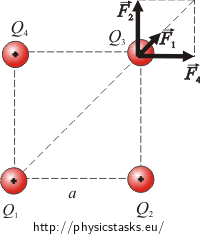• #### b) Analysis

We have to find the resulting electric forces caused by charges located at the corners of the square on the other charges before we calculate the value of charge q which has to be placed into the centre of the square in such a way so that the resultant force acting on charges in the corners is zero.

Charges placed at the corners of the square act upon the other charges with repulsive electric force. The size of these forces can be found from Coulomb’s law. The resulting force which acts on a single charge in the corner can be obtained by adding up the vectors of every force.

We have to place such a charge into the centre of the square so that it acts with the same force on the other charges but in the opposite direction. Since the charge in the centre of the square has to attract the other charges, it has to be negative. The value of this charge can be obtained from Coulomb’s law.

• #### b) Solution: Charge q Value

We will calculate the value of forces from Coulomb’s law: $F\,=\, k \, \frac{Q^2}{r^2}$

Only forces acting on charge Q3 are drawn into the picture. The others are analogical.Charges Q2 and Q4 are at distance a from charge Q3 and therefore they act on the charge with the same force:

$F_2\,=\,F_4\,=\, k \, \frac{Q^2}{a^2}$

The distance between Q1 and Q3 is $$a\sqrt{2}$$ which is the diagonal in a square with the side length of a.

$F_1\,=\, k \, \frac{Q^2}{\left( \sqrt{2} a \right)^2}$ $F_1\,=\, k \, \frac{Q^2}{2 a^2}$

We will obtain the resultant of acting forces by adding up their vectors. We will add up forces $$\vec{F}_2$$ and $$\vec{F}_4$$ first. The resultant of these two forces will be the diagonal in a square with the side length of F2 and its value will thus be: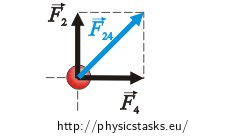$F_{24}\,=\,\sqrt{2}F_2\,.$ $F_{24}\,=\,\sqrt{2}k \, \frac{Q^2}{a^2}$

The resulting force $$\vec{F}$$ can be obtained by adding up the vectors of forces $$\vec{F}_{24}$$ and $$\vec{F}_1$$ whose values have been expressed.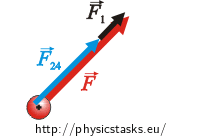$F\,=\,F_{24}+F_1$ $F\,=\,\sqrt{2}k \, \frac{Q^2}{a^2}\,+\,k \, \frac{Q^2}{2 a^2}$ $F\,=\,k \, \frac{Q^2}{a^2}\,\left(\sqrt{2}\,+\, \frac{1}{2 }\right)\tag{*}$

We have to place a charge at the centre of the square so that it will act with force of the same value $$\vec{F^,}$$ but in the opposite direction. We can find its value from Coulomb’s law.$F^,\,=\, k \,\frac{Q q}{\left(\frac{a\sqrt{2}}{2}\right)^2}\,,$

where $$\frac{a\sqrt{2}}{2}$$ is the half of the diagonal.

We will adjust the denominator of the fraction:

$F^,\,=\, k \,\frac{Q q}{\frac{a^2 2}{4}}\,=\, k \,\frac{Q q}{\frac{a^2}{2}}$ $F^,\,=\, k \,\frac{2Q q}{a^2}\tag{**}$

Now we will compare the expression of F and F , from relations (*) and (**):

$k \,\frac{2Q q}{a^2}\,=\, \,k \, \frac{Q^2}{a^2}\,\left(\sqrt{2}\,+\, \frac{1}{2 }\right)$

We will adjust and then express the searched charge q:

$2q \,=\,Q\,\left(\sqrt{2}\,+\, \frac{1}{2 }\right)$ $2q \,=\,Q\,\left(\frac{2\sqrt{2}\,+\, 1}{2 }\right)$ $q\,=\,Q\,\left(\frac{2\sqrt{2}\,+\, 1}{4 }\right)$

The electric intensity of the field is zero at the centre.

Potential at the centre of the square is equal to:

$\varphi_c\,=\,4 \varphi\,=\, \frac{1}{4 \pi \varepsilon_0}\, \frac{4\sqrt{2}Q}{a}\,.$

We have to place a negative charge of value:

$q\,=\,Q\,\left(\frac{2\sqrt{2}\,+\, 1}{4 }\right)\,.$

at the centre of the square so that the resultant force acting on the charges in the corners would be zero.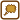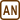×Original source: Diplomová práce Lenky Matějíčkové (2010).